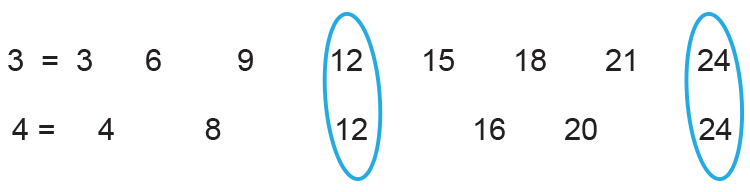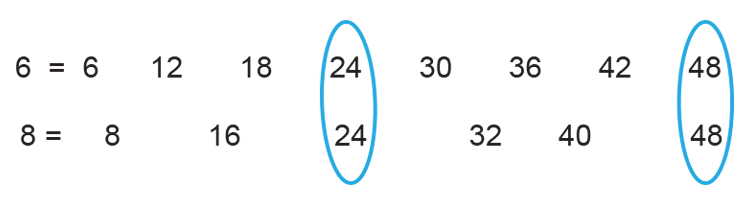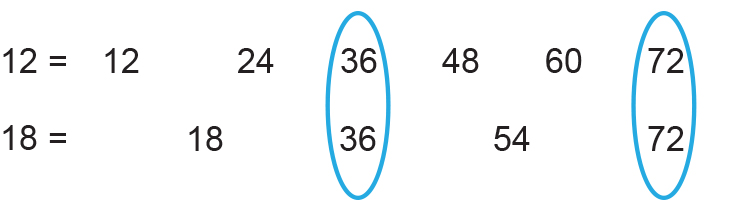# Lowest common multiple (LCM)

We know         Lowest = Smallest number

Common = Anything the same

Multiple = Multiplication table

Multiple here is short for
Multiplication tables

So logically

Lowest common multiple is

LCM - The smallest number
that they have in common
from multiplication tables

Example 1

What is the lowest common multiple of the two numbers 3 and 4?

Lowest common multiple:

1. The smallest number
2. What they have in common
3. From multiplication tables

Multiplcation tablesWhat they have in common are:

12 and 24

The smallest number is 12.

The lowest common multiple of the two numbers 3 and 4 is 12.

Example 2

What is the lowest common multiple of the two numbers 6 and 8?

Lowest common multiple:

1. The smallest number
2. What they have in common
3. From multiplication tables

Multiplcation tablesWhat they have in common are:

24 and 48

The smallest number is 24.

The lowest common multiple of the two numbers 6 and 8 is 24.

Example 3

What is the lowest common multiple of the two numbers 18 and 12?

Lowest common multiple:

1. The smallest number
2. What they have in common
3. From multiplication tables

Multiplcation tablesWhat they have in common is:

36 and 72

The smallest number is 36.